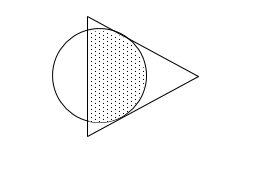# Question 1 of 8

The figure shown below is made up of a triangle and a circle. The area of the triangle is $\frac{3}{4}$ of the area of the circle. Given that $\frac{3}{5}$ of the circle is shaded, what fraction of the figure is shaded?A
$\frac{12}{23}$
B
$\frac{12}{26}$
C
$\frac{14}{23}$
D
$\frac{14}{26}$
E
None of the above